• Save

# Clock Problems Formula for SSC and Bank Exams

Last month 12.0K ViewsHere are given clock problems formulas for those students who are preparing for competitive exams. Clock problems are related to reasoning sections. As well as you can easily understand, how to use formulas in the clock problems with given short tricks.

These tricks will help you to solve clock problems in the competitive exam in less time. So, you should understand and practice these clock aptitude questions to obtain a better score in the competitive exam.

## Clock Problems Formula and Tricks

### What is clock problem?

Though we all know how to read time on clock, yet solution of question on clock requires some level of intelligence which is above on common level. We have laid down properties of clock with illustrative example so that students may not find any difficulty while solving question given in the exercise.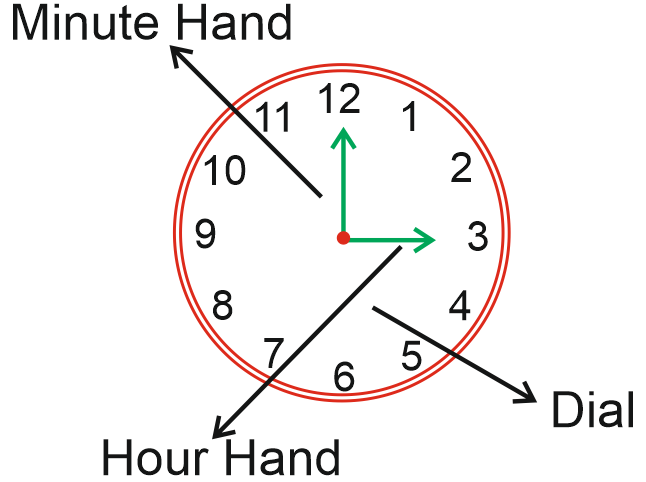The dial of the clock is numbered from 1 to 12 in such a way that each subsequent number is equidistant ( 5 min spaces apart) from the preceding number. It has two needle known as minute hand and hour hand. The distance between two consecutive number is 5 min. It means that the circumference of the clock measures 60 min space. When hour hand travels 5 min spaces, the minute hand travels 5 min spaces, the minute hand travels 60 min spaces. In this way, by travelling 60 min spaces, the minute hand gains 55 min on the hour hand.

Important Formula and Tips

1. In 60 min, the minute hand gains 55 min on the hour hand.

2. A clock is said to be too fast when it shows time more than that of shown by a correct clock. Likewise, a clock is said to be too slow when it shows time less than that shown by a correct clock.

3. Both the hands of clock are at right angle (When the distance between two hands measure 90) when they are 15 min space apart. This situation occurs once in a hour.

4. The hands of the clock are in the same straight line when they are coincident or opposite to each other.

5. In each hour only once both hands of a clock (minute and hour hand) face same direction and also only one time they face opposite direction. When both hands are opposite to each other the gap is of 30 min between them.

6. In a minute the hour hand moves by 0.50 and the minute hand moves by 60.

7. In each hour the minute hand covers more distance of 55 min in comparison to the hour hand.

8. Calculation of angle between the hands of clock.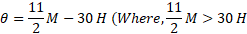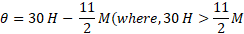Examples:

Ex.1. It is 4 hrs. 40 min. in a clock. Find the angle between both the needles of a clock.

(A) 1000

(B) 800

(C) 1200

(D) 2000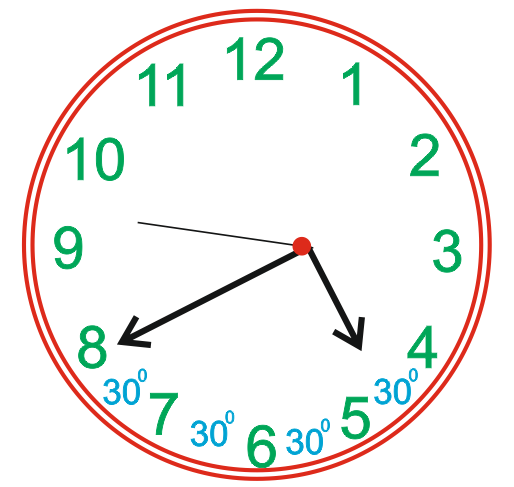1 Division = 300

So, 4 Divisions = 30 × 4 = 1200

But the hour needle has already covered the distance of 40 min. from its side, so the angle covered in these 40 min.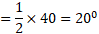Now, the angle covered between both needles = 1200 – 200 = 1000

Short Trick

To find the angle =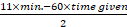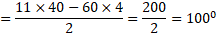Example.2. At what time between 7 and 8 0’clock will the hands of a clock be in the same straight line, but not together?

(A) 5 min past 7

(B)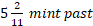(C)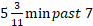(D)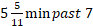Solution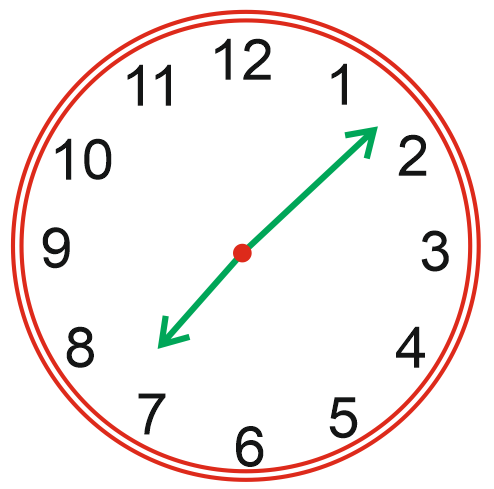Now as per properties of the clock,

Minute hand gains 55 min space in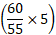min or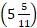min.

Therefore, the hands are in the same straight line, but not together at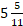min past 7.

Example.3. What will be the angle formed between two needles of a clock at 8 hrs. 20 min ?

(A) 1300

(B) 1400

(C) 1200

(D) 700

Solution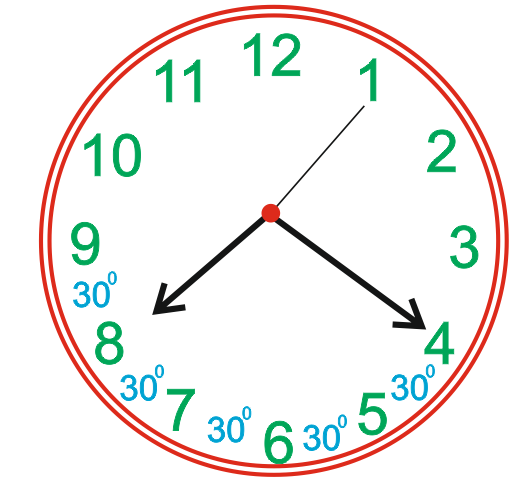Total division between both needles = 4

So, angle = 4×30 = 1200

But the hour needle has already covered the distance of 20 min. So, the angle covered by the hour needle in 20 min.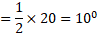Thus, the angle formed between both needles = 120+10 = 1300

Short Trick

To find the angle =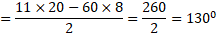Ex.4. At what time between 5:30 and 6 0’clock will the hands of clock be at right angle?

(A)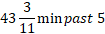(B)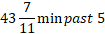(C) 40 min past 5

(D) 45 min past 5

Solution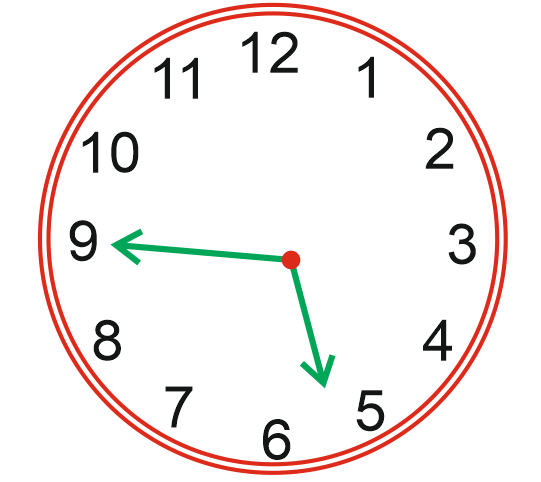55 min space is gained in 60 min.

Therefore, 40 min space will be gained in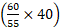min or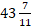Therefore, the hands are at right angle atmin pass 5.

Ex.5. A clock rings for once at 1.00 o’clock, for twice at 2.00 o’clock & so on at every hour. For how many times in a day it rings?

(A) 156

(B) 78

(C) 200

(D) 180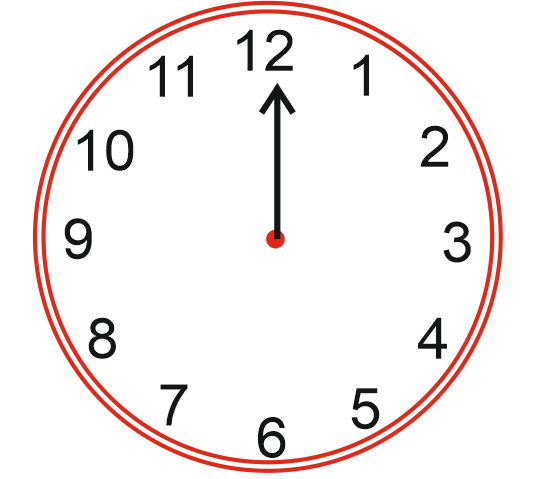Total hours in a day = 12. So, it will ring in 12 hours for :

1+2+3+4+5+6+7+8+9+10+11+12=78 times

So, in 24 hours, it will ring for 2 × 78 = 156 times

Short Trick

Sum from 1 to 12 =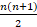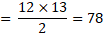So, in 24 hours, it will ring for 2 × 78 = 156.

Plz ask in the comment section if you face any problem regarding clock problems formulas.